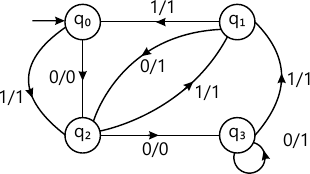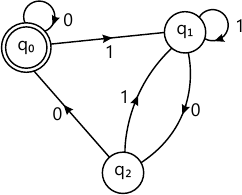MORE IN Theory of Computation
SPPU Information Technology (Semester 5)
Theory of Computation
May 2015
Total marks: --
Total time: --
INSTRUCTIONS
(1) Assume appropriate data and state your reasons
(2) Marks are given to the right of every question
(3) Draw neat diagrams wherever necessary

Answer any one question from Q1 and Q2
1 (a) Construct Moore machine equivalent for the given Mealy machine.6 M
1 (b) Let ∑={a,b}. Write RE to define language consisting of strings such that
i) String without substring bb
ii) String that have exactly one double letter in them.
4 M

2 (a) Design a DFA for accepting L over {0,1} such that every substring of length 4 contains at least three 1's.
4 M
2 (b) Define Finite Automata and justify why palindrome strings cannot be checked for by FSM.
4 M
2 (c) With examples define Regular Expression.
2 M

Answer any one question from Q3 and Q4
3 (a) Construct NFA accepting language represented by 0*1*2* and convert it into DFA.
6 M
3 (b) Find RE for the following DFA using Arden?s theorem.4 M

4 (a) Give the CFG for *sum;={a,b}. i) To generate strings in which no consecutive b's can occur but a's can be consecutive. ii) Language is {ax by /x ≠ y and x,y > 0}
4 M
4 (b) Convert the given grammar into GNF:
S → AB
A → BS|b
iii) B → SA|a.
4 M
4 (b) Write a note on applications of CFG.
2 M

Answer any one question from Q5 and Q6
5 (a) Construct a PDA to accept language {anbmC(n+m)where n, m>=1}.
6 M
5 (b) Compare PDA and FA.
4 M

6 (a) Construct a post m/c to accept the language {anbn+1/where n>=1}
8 M
6 (b) Construct a CFG equivalent to PDA. [ M=left ( { q_0, q_1}, {0, 1}, {B,R}, {delta, q_0, R, phi} ight ) where delta is \ delta (q_0, o, R) = (q_0, BR)\ delta (q_0, o B)= (q_0, BB) \ delta (q_0, 1, B)= (q_1, B) \ delta (q_1, 1,B)= (q_1, B) \ delta (q_1, 0B)= (q_1, epsilon) \ delta ( q_1 , wedge , R) = (q_1, varepsilon) ]
8 M
6 (c) Define Post Machine.
2 M

Answer any one question from Q7 and Q8
7 (a) Design a TM that computes the function [ egin {align*} f(x,y)&=0 x+y & if x> = y \ &=0 & if x Simulate the working of the TM for x=2, y=2.
12 M
7 (b) Explain the different types of Turing machines.
4 M

8 (a) Define Turing Machine and construct a TM which recognizes strings consisting of equal number of 0's and 1's.
8 M
8 (b) Compare FA, PDA and TM.
4 M
8 (c) Explain the halting problem of Turing machines.
4 M

Answer any one question from Q9 and Q10
9 (a) Explain with example Turing Reducibility.
6 M
9 (b) Prove that the following decision problems are recursive.
i) Two DFA's are equivalent or Not.
ii) NFA accepts a word or not.
10 M

10 (a) Define and differentiate recursive language and recursively enumerable languages.
6 M
10 (b) P.T. the following decision problems are recursive
i) DFA accepts a word or not
ii) CFG G generates the string w or not.
10 M

More question papers from Theory of Computation